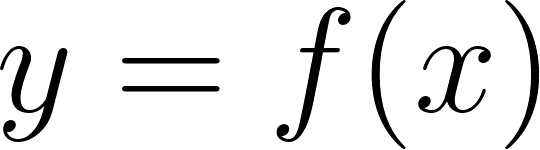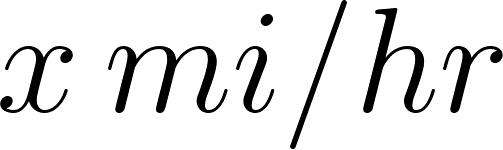×
Get Full Access to Calculus: Early Transcendentals - 1 Edition - Chapter 1.2 - Problem 46e
Get Full Access to Calculus: Early Transcendentals - 1 Edition - Chapter 1.2 - Problem 46e

×

Answer: Functions from words Find a formula for a functionISBN: 9780321570567 2

Solution for problem 46E Chapter 1.2

Calculus: Early Transcendentals | 1st Edition

• Textbook Solutions
• 2901 Step-by-step solutions solved by professors and subject experts
• Get 24/7 help from StudySoup virtual teaching assistantsCalculus: Early Transcendentals | 1st Edition

4 5 1 286 Reviews
24
3
Problem 46E

Functions from words: Find a formula for a function describing the given situation. Graph the function and give a domain that makes sense for the problem. Recall that with constant speed distance = speed time elapsed or. A functionsuch that if you ride a bike formi atmiles per hour ,you arrive at your destination inhours.

Step-by-Step Solution:

Step 1 of 4

Given,Ride a bike formi atmiles per hour.

This means,

DistanceVelocity or speed atWe need to find a formula for a function describing the given situation.

Step 2 of 4

Step 3 of 4

ISBN: 9780321570567

Calculus: Early Transcendentals was written by and is associated to the ISBN: 9780321570567. This full solution covers the following key subjects: function, speed, functions, DES, Describing. This expansive textbook survival guide covers 85 chapters, and 5218 solutions. The answer to “?Functions from words: Find a formula for a function describing the given situation. Graph the function and give a domain that makes sense for the problem. Recall that with constant speed distance = speed time elapsed or . A function such that if you ride a bike for mi at miles per hour ,you arrive at your destination in hours.” is broken down into a number of easy to follow steps, and 60 words. The full step-by-step solution to problem: 46E from chapter: 1.2 was answered by , our top Calculus solution expert on 03/03/17, 03:45PM. This textbook survival guide was created for the textbook: Calculus: Early Transcendentals, edition: 1. Since the solution to 46E from 1.2 chapter was answered, more than 398 students have viewed the full step-by-step answer.

Unlock Textbook Solution